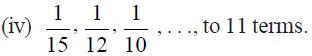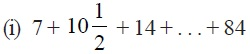Study Materials

# NCERT Solutions for Class 10th Mathematics

Page 3 of 4

## Chapter 5. Arithmetic Progressions

### Exercise 5.3

Exercise 5.3

Q1. Find the sum of the following APs:
(i) 2, 7, 12, . . ., to 10 terms.

(ii) –37, –33, –29, . . ., to 12 terms.
(iii) 0.6, 1.7, 2.8, . . ., to 100 terms.Q2. Find the sums given below :(ii) 34 + 32 + 30 + . . . + 10
(iii) –5 + (–8) + (–11) + . . . + (–230)
Q3. In an AP:
(i) given a = 5, d = 3, an = 50, find n and Sn.
(ii) given a = 7, a13 = 35, find d and S13.
(iii) given a12 = 37, d = 3, find a and S12.
(iv) given a3 = 15, S10 = 125, find d and a10.
(v) given d = 5, S9 = 75, find a and a9.
(vi) given a = 2, d = 8, Sn = 90, find n and an.
(vii) given a = 8, an = 62, Sn = 210, find n and d.
(viii) given an = 4, d = 2, Sn = –14, find n and a.
(ix) given a = 3, n = 8, S = 192, find d.
(x) given l = 28, S = 144, and there are total 9 terms. Find a.

Q4. How many terms of the AP : 9, 17, 25, . . . must be taken to give a sum of 636?
Q5. The first term of an AP is 5, the last term is 45 and the sum is 400. Find the number of terms and the common difference.
Q6. The first and the last terms of an AP are 17 and 350 respectively. If the common difference is 9, how many terms are there and what is their sum?
Q7. Find the sum of first 22 terms of an AP in which d = 7 and 22nd term is 149.
Q8. Find the sum of first 51 terms of an AP whose second and third terms are 14 and 18 respectively.
Q9. If the sum of first 7 terms of an AP is 49 and that of 17 terms is 289, find the sum of first n terms.

Page 3 of 4

Chapter Contents: